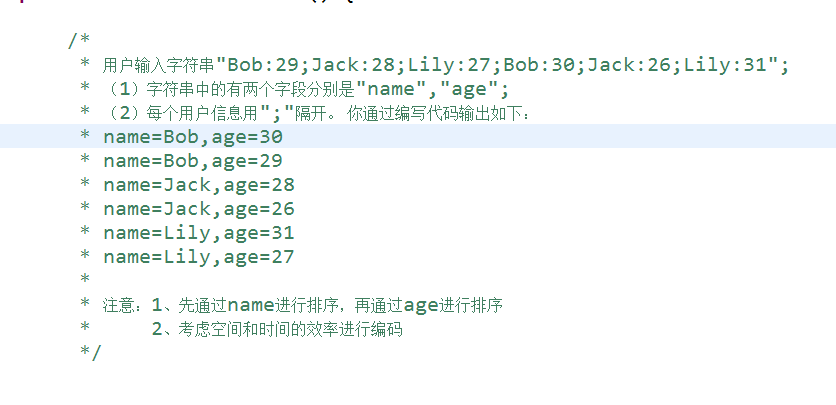（1）字符串中的有两个字段分别是"name","age";
（2）每个用户信息用";"隔开。

name=Bob,age=30
name=Bob,age=29
name=Jack,age=28
name=Jack,age=26
name=Lily,age=31
name=Lily,age=27

2、考虑空间和时间的效率进行编码

（望大家帮忙）

1个回答

public static void main(String[] args) {
String str = "Bob:29;Jack:28;Lily:27;Bob:30;Jack:26;Lily:31";
String[] strs=str.split(";");
Arrays.sort(strs);
for(String s:strs){
System.out.println("name="+s.substring(0,s.indexOf(":"))+",age="+s.substring(s.indexOf(":")+1));
}
}

name=Bob,age=29
name=Bob,age=30
name=Jack,age=26
name=Jack,age=28
name=Lily,age=27
name=Lily,age=31